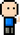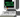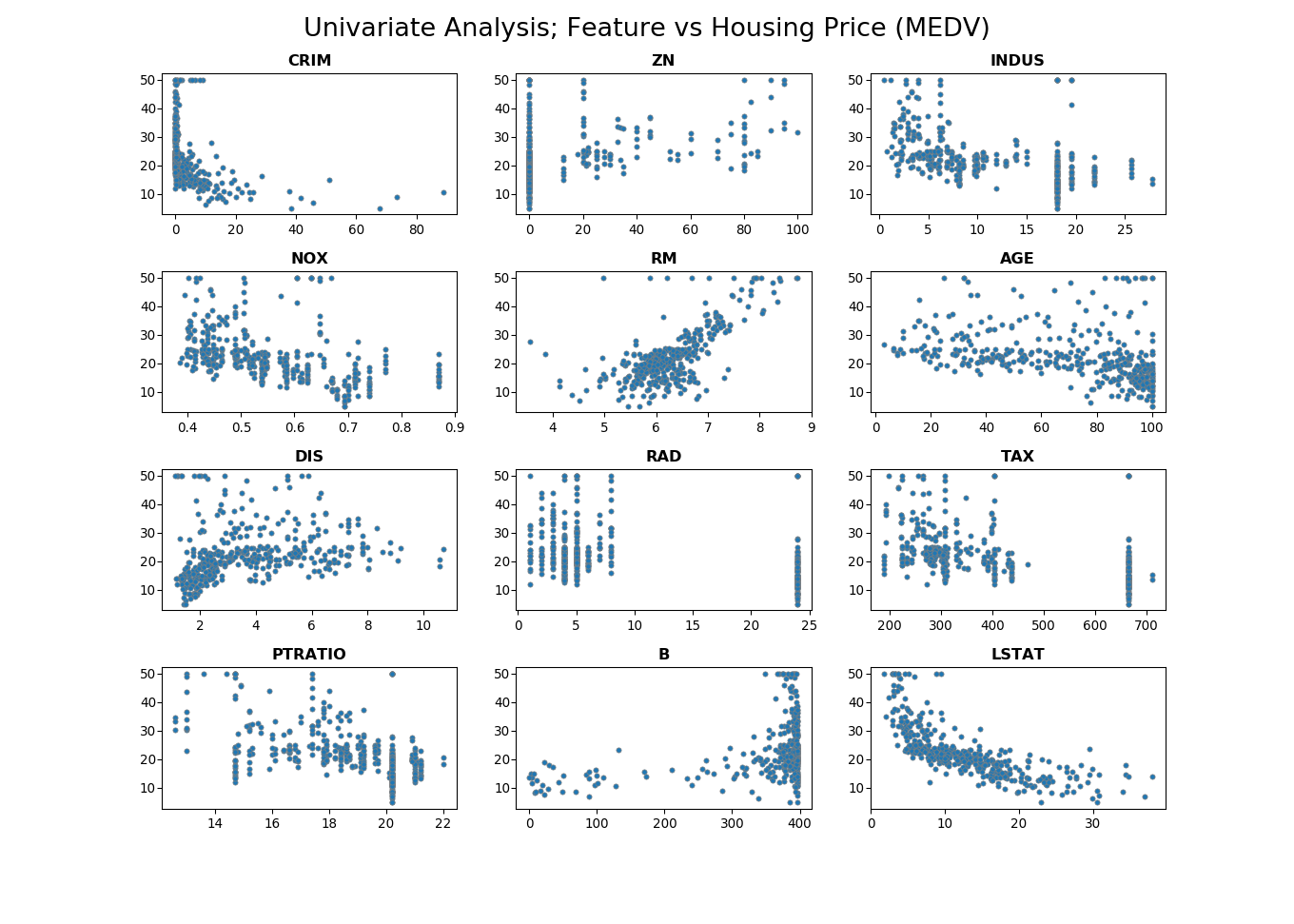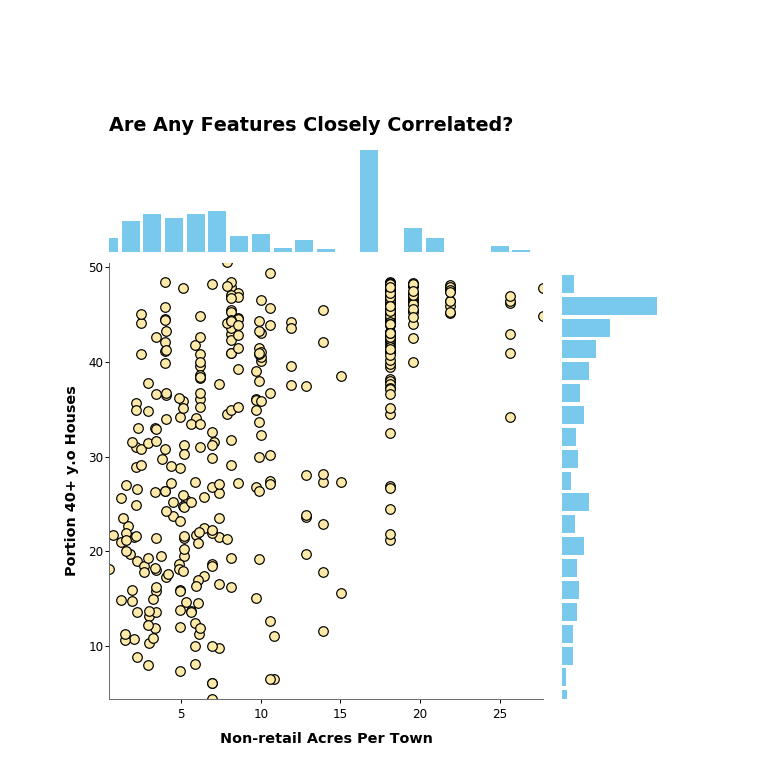# Josh Meets Computer# Analysis and Regression model

April 11, 2018

What does an Engineer need to do to deploy a Machine Learning model to a software application? There is a lot that into a full pipeline — from aggregating the data from source, cleaning, pre-processing and transforming to a model-friendly input, training the model to the point of generating accurate predictions, then maybe even deploying the trained model (and possibly the pre-processing algorithms) as a function call in a software application. Even in that list there can be more steps.

In the walk through below I take a popular research dataset and show the steps a Software Engineer might take to build and deploy a machine learning powered application.

## Libraries and Visualization Parameters

``````import pandas as pd
import numpy as np
import matplotlib.pyplot as plt
import matplotlib

from matplotlib import gridspec
from keras.datasets import boston_housing
from sklearn import preprocessing as p
from sklearn import model_selection as mdl
from keras.models import Sequential
from keras.layers import Dense``````
``Using TensorFlow backend.``
``````# Visualization settings
%matplotlib inline
matplotlib.rcParams['figure.figsize'] = (16, 10)
matplotlib.rcParams['ytick.major.pad']='1'
plt.rcParams['axes.labelweight'] = 'bold'
plt.rcParams["axes.labelsize"] = 15

# Print Settings
pd.set_option('display.width', 2000)
pd.set_option('precision', 3)
np.set_printoptions(precision=3, suppress=True)``````

## The Dataset

I’ll be working with the Boston Housing dataset, downloadable straight from the Keras Machine Learning library. The data comes in as numpy arrays, but the associated columns are as follows:

``````CRIM - per capita crime rate by town
ZN -proportion of residential land zoned for lots over 25,000 sq.ft.
INDUS - proportion of non-retail business acres per town.
CHAS - Charles River dummy variable (1 if tract bounds river; 0 otherwise)
NOX - nitric oxides concentration (parts per 10 million)
RM - average number of rooms per dwelling
AGE - proportion of owner-occupied units built prior to 1940
DIS - weighted distances to five Boston employment centres
RAD - index of accessibility to radial highways
TAX - full-value property-tax rate per 10,000
PTRATIO - pupil-teacher ratio by town
B - 1000(Bk - 0.63)^2 where Bk is the proportion of blacks by town
LSTAT - Percent lower status of the population
MEDV - Median value of owner-occupied homes in 1000's``````

This dataset is used to describe the median value of owner-occupied homes during the 1980’s. The goal is to build a regression model that predects the expected median value based on the thirteen features provided in the dataset.

``````(x_train, y_train), (x_test, y_test) = boston_housing.load_data()
print('Data loaded. Rows: {}, Columns: {}'.format(*x_train.shape))``````
``Data loaded. Rows: 404, Columns: 13``

## Exploring the Data

This dataset is quite small, which will probably not give us a super accurate model, but at least it’s easy to work with. With the dataset in hand, time to do some exploring. I am interested in things like missing values, properly formatted data points, spread, outliers and relationships in the data.

``````x_headers = ["CRIM", "ZN","INDUS", "CHAS", "NOX", "RM", "AGE", "DIS", "RAD", "TAX", "PTRATIO", "B", "LSTAT"]
y_headers = ["MEDV"]

print('Number of zero values per column:\n')
_ = [print(h, end='\t') for h in x_headers]
print()
trainDF = pd.DataFrame(x_train, columns=x_headers)
for h in x_headers:
print((trainDF[h] == 0).sum(), end='\t')
trainDF.head()``````
``````Number of zero values per column:

CRIM	ZN	INDUS	CHAS	NOX	RM	AGE	DIS	RAD	TAX	PTRATIO	B	LSTAT
0	300	0	379	0	0	0	0	0	0	0	0	0``````
CRIM ZN INDUS CHAS NOX RM AGE DIS RAD TAX PTRATIO B LSTAT
0 1.232 0.0 8.14 0.0 0.538 6.142 91.7 3.977 4.0 307.0 21.0 396.90 18.72
1 0.022 82.5 2.03 0.0 0.415 7.610 15.7 6.270 2.0 348.0 14.7 395.38 3.11
2 4.898 0.0 18.10 0.0 0.631 4.970 100.0 1.333 24.0 666.0 20.2 375.52 3.26
3 0.040 0.0 5.19 0.0 0.515 6.037 34.5 5.985 5.0 224.0 20.2 396.90 8.01
4 3.693 0.0 18.10 0.0 0.713 6.376 88.4 2.567 24.0 666.0 20.2 391.43 14.65

## Observations

• Whether a house borders the charles river is a boolean value, and most are set to 0.
• The proportion of residential land zoned for lots over 25,000sq-ft is often 0%.
• No missing values in the dataset, woot woot!
``````fig, axes= plt.subplots(4, 3)
_ = pd.DataFrame(x_train, columns=x_headers).drop('CHAS',1).plot(kind='box',
subplots=True,
ax=axes,
showfliers=False,
vert=False,
colormap='jet')

_ = fig.suptitle("Spread of Features",fontsize=20)``````
``````fig, axes= plt.subplots(4, 3)
_ = pd.DataFrame(p.scale(x_train), columns=x_headers).drop('CHAS',1).plot(kind='hist',
bins=20,
subplots=True,
ax=axes)

_ = fig.suptitle("Distribution of Scaled Features",fontsize=20)

for ax in axes.flat: ax.set_ylabel('')``````
``````fig, axes= plt.subplots(4, 3, figsize=(14,10))

_ = fig.suptitle('Univariate Analysis; Feature vs Housing Price (MEDV)', fontsize=20)

df = pd.DataFrame(x_train, columns=x_headers).drop('CHAS',1)
headers = df.columns
i = 0

for ax in axes.flat:
ax.set_title(headers[i], fontweight='bold', fontsize=12)
ax.scatter(df.iloc[:,i], y_train, s=15, marker='o', edgecolor='grey', linewidth='0.5')
i += 1

plt.subplots_adjust(top=0.92, hspace=0.4)``````
``<IPython.core.display.Javascript object>``## Observations

• LSTAT seems like an inverse log relationship to Median Housing Price
• The only feature with a nicely distributed range of values is average number of rooms. The rest seem skewed
• Property Tax rate looks nearly categorical
• accessibility to highways looks pretty categorical
• Room count is correlated to housing price
• Percent lower status of the population is inversely correlated to housing price

### Are any two features highly correlated?

A common statistical technique to perform on a new dataset is multivariate analysis. This is to compare the features of a dataset in order to discover relationships in the data, especially when there may be more than one independent variable and more than one dependent variable. It can be a useful way to hypothesize if the output (median value of houses) is affected by certain variables (input). There’s a lot to explore in this space, but below is a run through of all the variables of the dataset.

``````%reload_ext autoreload
from dataviz import graph_animator
from matplotlib import rc
from IPython.display import HTML
%matplotlib notebook

rc('animation', html='html5')
viz = graph_animator()``````
``````Traceback (most recent call last):
File "C:\Users\joshu\Anaconda3\envs\MLenv\lib\site-packages\matplotlib\cbook\__init__.py", line 388, in process
proxy(*args, **kwargs)
File "C:\Users\joshu\Anaconda3\envs\MLenv\lib\site-packages\matplotlib\cbook\__init__.py", line 228, in __call__
return mtd(*args, **kwargs)
File "C:\Users\joshu\Anaconda3\envs\MLenv\lib\site-packages\matplotlib\animation.py", line 1499, in _stop
self.event_source.remove_callback(self._loop_delay)
AttributeError: 'NoneType' object has no attribute 'remove_callback'

<IPython.core.display.Javascript object>``````## Further Data Analysis

These visualizations are just a couple tools used to better understand the data. From here, we can take a closer look at each feature and see how it contributes to the median house price value. We can figure out if new features can be engineered from the data; perhaps passing LSTAT through a log function could create a better predictive Model. Ultimately the techniques around statistical data analysis should help a researcher become more familiar with the properties of the data.

## Creating a Model

The chosen model architecture for this problem was a two layer neural network with a mean squared error loss function. Below is the implementation, which includes the creation of the architecture, calculation of the loss function, as well as the training algorithm.

``````class NeuralNetwork():
"""
Two (hidden) layer neural network model.
First and second layer contain the same number of hidden units
"""
def __init__(self, input_dim, units, std=0.0001):
self.params = {}
self.input_dim = input_dim

self.params['W1'] = np.random.rand(self.input_dim, units)
self.params['W1'] *= std
self.params['b1'] = np.zeros((units))

self.params['W2'] = np.random.rand(units, units)
self.params['W2'] *= std * 10  # Compensate for vanishing gradients
self.params['b2'] = np.zeros((units))

self.params['W3'] = np.random.rand(units, 1)
self.params['b3'] = np.zeros((1,))

def mse_loss(self, x, y=None, drop_p=0.9, reg=0.01, evaluate=False, predict=False):

# Unpack variables
W1, b1 = self.params['W1'], self.params['b1']
W2, b2 = self.params['W2'], self.params['b2']
W3, b3 = self.params['W3'], self.params['b3']
N, D = x.shape

###############################################
# Forward Pass
###############################################
Fx = None

# First Layer
x1 = np.dot(x, W1) + b1

# Activation
a1 = np.maximum(x1, 0)

# Drop Out
drop1 = np.random.choice([1,0],size=x1.shape, p=[drop_p, 1-drop_p]) / drop_p
a1 *= drop1

# Second Layer
x2 = np.dot(a1, W2) + b2

# Activation
a2 = np.maximum(x2, 0)

# Drop Out
drop2 = np.random.choice([1,0], size=x2.shape, p=[drop_p, 1-drop_p]) / drop_p
a2 *= drop2

# Final Layer
x3 = np.dot(a2, W3) + b3

# Output
Fx = x3

if predict:
return Fx

# Mean Squared Error Cost Function
mse_loss = np.sum((Fx - y.reshape(-1,1))**2, axis=0) / N
mae_loss = np.sum(np.absolute(Fx - y.reshape(-1,1)), axis=0) / N
wght_loss = 0.5 * reg * (np.sum(W1**2) + np.sum(W2**2) + np.sum(W3**3))
loss = mse_loss + wght_loss

if evaluate:
return {'loss':loss,
'mean_absolute_error': mae_loss,
'mean_squared_error': mse_loss,
'weight_loss': wght_loss}

#############################################
# Backpropagation
#############################################

grads = {}

# Output
dFx = 2 * (Fx.copy() - y) / N  # [50, 1]

# Final Layer
dx3 = np.dot(dFx, W3.T)
dW3 = np.dot(x2.T, dFx)
db3 = np.sum(dFx * N, axis=0)

# Drop Out
dx3 *= drop2

# activation
da2 = a2.copy()
da2[da2 > 0] = 1
da2[da2 <= 0] = 0
da2 *= dx3

# Second Layer
dx2 = np.dot(da2, W2.T)
dW2 = np.dot(x1.T, da2)
db2 = np.sum(da2, axis=0)

# Drop out
dx2 *= drop1

# activation
da1 = a1.copy()
da1[da1 > 0] = 1
da1[da1 < 0] = 0
da1 *= dx2

# First Layer
dx1 = np.dot(da1, W1.T)
dW1 = np.dot(x.T, da1)
db1 = np.sum(da1, axis=0)

grads['W3'] = dW3
grads['b3'] = db3
grads['W2'] = dW2
grads['b2'] = db2
grads['W1'] = dW1
grads['b1'] = db1

grads['W3'] += dW3 * reg
grads['W2'] += dW2 * reg
grads['W1'] += dW1 * reg

return mae_loss, loss, grads

def fit(self, X, y, validation_data, epochs=80,
learning_rate=1e-3, learning_rate_decay=0.99,
reg=1e-5, batch_size=50, dropout_val=0.95):

assert type(validation_data) == tuple
x_val, y_val = validation_data

num_train = X.shape
iters_per_epoch = max(num_train // batch_size, 1)
val_acc = 0

loss_history = []
val_loss_history = []
mae_history = []
val_mae_history = []

for e in range(epochs):
for it in range(iters_per_epoch):
x_batch = None
y_batch = None

batch_indices = np.random.choice(num_train,
batch_size,
replace=False)

x_batch = X[batch_indices]
y_batch = y[batch_indices]

mae, loss, grads = self.mse_loss(x_batch,
y_batch,
drop_p=dropout_val,
reg=reg)

val_mae, val_loss, _ = self.mse_loss(x_val, y_val)

for key in self.params:
self.params[key] -= learning_rate * grads[key]

if it % iters_per_epoch == 0:
learning_rate *= learning_rate_decay

# Record cost values for this epoch
loss_history.append(loss)
mae_history.append(mae)
val_loss_history.append(val_loss)
val_mae_history.append(val_mae)

return {'loss': loss_history,
'mean_absolute_error': mae_history,
'val_loss': val_loss_history,
'val_mean_absolute_error': val_mae_history}

def evaluate(self, X, y):
return self.mse_loss(X, y, drop_p=1, evaluate=True)

def predict(self, X):
return self.mse_loss(X, drop_p=1, predict=True)``````

## Base Model Notes

If the dataset is small, then there won’t be enough samples to be statistically representative of the data at hand. Few data points means the model will be very prone to overfitting, and an overly complex model with a large architecture will optimize very well to the training data but will be too finely tuned to be able to generalize to new examples. For this reason, the model was built as a two layer neural network. Relu activations were included for predicting with non-linearities.

The loss function / optimization function on this model is the Mean Squared Error, which calculates the distance between the predicted value and the ground truth value, squares the difference then sums this value across all examples. Squaring the difference will exponentially penalize inaccurate estimates, creating a steeper gradient descent in initial training.

The model will be measured by the mean absolute error. This is just the absolute difference between the predicted value and the actual value, translates to actual dollar difference between the prediction and the actual median cost of housing.

## Pre-Training Step: Visualization Helper

``````class plot_handler():
"""
' Plot handler to help me control the shape of my subplots better.
"""
def __init__(self, plot_rows, plot_cols):
self.rows = plot_rows
self.cols = plot_cols
self.fig = plt.figure(facecolor='white', figsize=(16,16))
self.grid = gridspec.GridSpec(self.rows, self.cols)

self.grid.update(left=0.1,
right=0.9,
wspace=0.2,
hspace=.15,
top=0.9,
bottom=0.1)

self.ax = {}
self.xlimit = None
self.ylimit = None

def __call__(self):
plt.show

def add_plot(self, top, bottom, left, right, name, title):
self.ax[name] = self.fig.add_subplot(self.grid[top:bottom, left:right])
self.ax[name].set_title(title,fontweight="bold", size=14)

# self.ax[name].set_xticks([])
# self.ax[name].set_yticks([])
def plot_exists(self, name):
if name in self.ax:
return True
else:
return False

def plot(self, data, plot_name, ylim=None, c='b', alpha=1.0):
self.ax[plot_name].plot(data,  '-', c=c, alpha=alpha)

if not ylim:
self.ax[plot_name].set_ylim([0,ylim])``````

## Training and validating the model using K-folds

K-fold validation is the technique of splitting your data during training and averaging the results of a model trained on the seperate sets. The process goes like this: split your data into k segments. Create k batches of data, where for each batch, the training set has a unique k-1 segments and the validation set is the remaining 1 segment. Train a new model on each of these batches (or folds), averaging the results.

Generally, if different shuffling of data before splitting into test and train yield a different model performance, then there is not enough data and k-folding would be a good approach.

## Pre-processing the data

Most predictive models work best under the assumption that the data is centered about zero, and that all features have an equal magnitude of variance. This equal variance allows the cost function of the model to weight all features equally. If some features are more important than others, they can be scaled differently so that the features variability contributes more to the cost function. Because of this, it’s a good idea to translate the dataset so it is centered around zero and scale each feature to have a unit standard deviation.

It’s worth noting that some models, like Decision Tree algorithms are robust to different scales.

``````import matplotlib.patches as mpatches

# join the x and y together for shuffling
y_train = y_train.reshape((y_train.shape,1))
data = np.hstack([x_train, y_train])

# Make random generation repeatable
seed = 7
np.random.seed(seed)

# small dataset, shuffling and folding the data
shuff_count, i = 3, 0
split_count = 4
epoch_count = 80
b = 0

# Fit a scaling function to the train data
scale = p.StandardScaler().fit(x_train)

# Plotting Parameters #####################
subplot = ['Mean Absolute Error', 'Loss'] * 2
metrics = ['mean_absolute_error', 'loss', 'val_mean_absolute_error', 'val_loss']
legends = ['Train', 'Train', 'Validation', 'Validation']

history_set = {metric: np.zeros(shape=(epoch_count, shuff_count * split_count)) for metric in metrics}

plotter = plot_handler(2, 1)
plotter.add_plot(top=0,bottom=1,left=0,right=1,
name='Loss',
title='Average Loss')
plotter.add_plot(top=1,bottom=2, left=0, right=1,
name='Mean Absolute Error',
title='House Price Error: Estimate vs Actual (in \$1000s)')
############################################

best_model = NeuralNetwork(input_dim=x_train.shape, units=64)  # base_model()

while i < shuff_count:
i += 1
data_folds = mdl.KFold(n_splits=split_count,
shuffle=True,
random_state=seed).split(data)

for dfold in data_folds:
train, valid = dfold

# Seperate each k fold of train data into a train and validation set
xt = scale.transform(data[train,:-1])
yt = data[train,-1:]

# Transform validation set based on scaling on training set
xv = scale.transform(data[valid,:-1])
yv = data[valid,-1:]

model = NeuralNetwork(input_dim=x_train.shape, units=64)  # base_model()
history = model.fit(xt, yt, validation_data=(xv, yv), epochs=epoch_count)

for m in metrics:
# history_set[m][:, b] = history.history[m]
history_set[m][:, b] = history[m]

for metric, plot in zip(metrics, subplot):
color = 'blue' if  metric[:3] == 'val' else 'green'
plotter.ax[plot].plot(history[metric],
c=color,
linewidth=2,
alpha=0.2)

# Evaluate each model, keep the best one
curr_evaluation = model.evaluate(scale.transform(x_test), y_test)  #, verbose=0)
best_evaluation = best_model.evaluate(scale.transform(x_test), y_test)  #, verbose=0)

if curr_evaluation['mean_squared_error'] < best_evaluation['mean_squared_error']:
best_model = model

b += 1

# Plot average of all folds
for metric, plot in zip(metrics, subplot):
color = 'blue' if metric[:3] == 'val' else 'green'
plotter.ax[plot].plot(np.mean(history_set[metric], axis=1),
c=color,
linewidth=3,
alpha=1.0)

# Add Legend
train = mpatches.Patch(color='green', label='Train')
valid = mpatches.Patch(color='blue', label='Validation')

for plot in subplot[:2]:
plotter.ax[plot].legend(handles=[train, valid])

plotter.ax['Loss'].set_ylim([0,100])
plotter.ax['Mean Absolute Error'].set_ylim([0,10])

plotter()``````
``<IPython.core.display.Javascript object>``